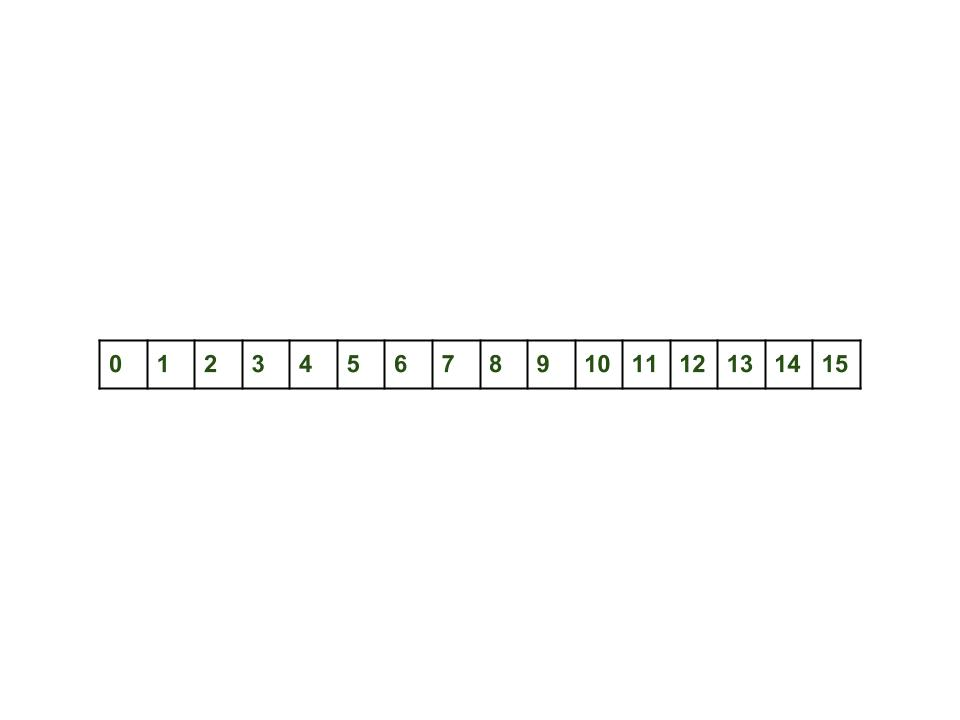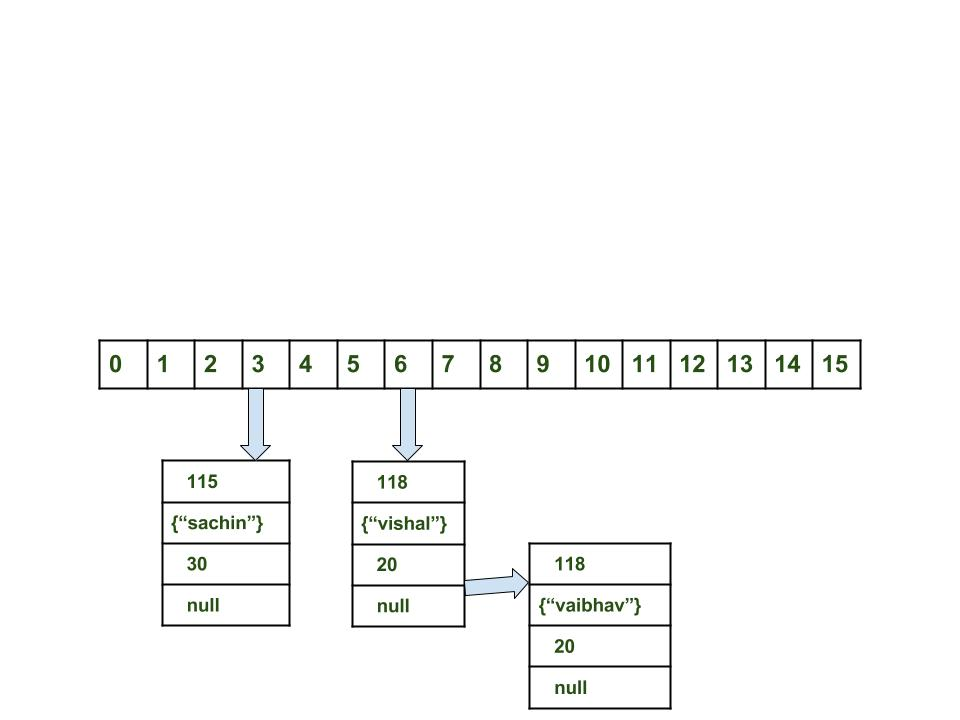Open In App

# Internal Working of HashMap in Java

In this article, we will see how the hashmap get and put method works internally. What operations are performed? How the hashing is done. How the value is fetched by key. How the key-value pair is stored.
In the previous article, HashMap contains an array of Node and Node can represent a class having the following objects :

1. int hash
2. K key
3. V value
4. Node next

Now we will see how this works. First, we will see the hashing process.

Hashing

Hashing is a process of converting an object into integer form by using the method hashCode(). It’s necessary to write the hashCode() method properly for better performance of HashMap. Here I am taking the key of my class so that I can override the hashCode() method to show different scenarios. My Key class is

```//custom Key class to override hashCode()
// and equals() method
class Key
{
String key;
Key(String key)
{
this.key = key;
}

@Override
public int hashCode()
{
return (int)key.charAt(0);
}

@Override
public boolean equals(Object obj)
{
return key.equals((String)obj);
}
}```

Here override hashCode() method returns the first character’s ASCII value as hash code. So whenever the first character of the key is same, the hash code will be the same. You should not approach these criteria in your program. It is just for demo purposes. As HashMap also allows a null key, so hash code of null will always be 0.

hashCode() method: hashCode() method is used to get the hash code of an object. hashCode() method of the object class returns the memory reference of an object in integer form. Definition of hashCode() method is public native hashCode(). It indicates the implementation of hashCode() is native because there is not any direct method in java to fetch the reference of the object. It is possible to provide your implementation of hashCode().
In HashMap, hashCode() is used to calculate the bucket and therefore calculate the index.

equals() method: This method is used to check whether 2 objects are equal or not. This method is provided by the Object class. You can override this in your class to provide your implementation.
HashMap uses equals() to compare the key to whether they are equal or not. If the equals() method return true, they are equal otherwise not equal.

Buckets: A bucket is an element of the HashMap array. It is used to store nodes. Two or more nodes can have the same bucket. In that case, a link list structure is used to connect the nodes. Buckets are different in capacity. A relation between bucket and capacity is as follows:

`capacity = number of buckets * load factor`

A single bucket can have more than one node, it depends on the hashCode() method. The better your hashCode() method is, the better your buckets will be utilized.

Index Calculation in Hashmap

The Hash code of the key may be large enough to create an array. hash code generated may be in the range of integer and if we create arrays for such a range, then it will easily cause outOfMemoryException. So we generate an index to minimize the size of the array. The following operation is performed to calculate the index.

`index = hashCode(key) & (n-1).`

where n is the number of buckets or the size of the array. In our example, I will consider n as the default size which is 16.

Why the above method is used to calculate the index

Using a bitwise AND operator is similar to doing bit masking wherein only the lower bits of the hash integer is considered which in turn provides a very efficient method of calculating the modulus based on the length of the hashmap.

• Initially Empty hashMap: Here, the hashmap’s size is taken as 16.

`HashMap map = new HashMap();`
• HashMap :• Inserting Key-Value Pair: Putting one key-value pair in the above HashMap

`map.put(new Key("vishal"), 20);`
• Steps:
1. Calculate hash code of Key {“vishal”}. It will be generated as 118.
2. Calculate index by using index method it will be 6.
3. Create a node object as :
```{
int hash = 118

// {"vishal"} is not a string but
// an object of class Key
Key key = {"vishal"}

Integer value = 20
Node next = null
}```
1. Place this object at index 6, if no other object is presented there.
• Inserting another Key-Value Pair: Now, putting the other pair that is,
`map.put(new Key("sachin"), 30);`
• Steps:
1. Calculate hashCode of Key {“sachin”}. It will be generated as 115.
2. Calculate index by using index method it will be 3.
3. Create a node object as :

```{
int hash = 115
Key key = {"sachin"}
Integer value = 30
Node next = null
}```
• In Case of collision: Now, putting another pair that is,

`map.put(new Key("vaibhav"), 40);`
• Steps:
1. Calculate hash code of Key {“vaibhav”}. It will be generated as 118.
2. Calculate index by using index method it will be 6.
3. Create a node object as :
``` {
int hash = 118
Key key = {"vaibhav"}
Integer value = 40
Node next = null
}```
1. Place this object at index 6 if no other object is presented there.
2. In this case, a node object is found at index 6 – this is a case of collision.
3. In that case, check via the hashCode() and equals() method if both the keys are the same.
4. If keys are the same, replace the value with the current value.
5. Otherwise, connect this node object to the previous node object via linked list and both are stored at index 6.
Now HashMap becomes :Using the get method()

Now let’s try some get methods to get a value. get(K key) method is used to get a value by its key. If you don’t know the key then it is not possible to fetch a value.

• Fetch the data for key sachin:
`map.get(new Key("sachin"));`
• Steps:
1. Calculate hash code of Key {“sachin”}. It will be generated as 115.
2. Calculate index by using index method it will be 3.
3. Go to index 3 of the array and compare the first element’s key with the given key. If both are equals then return the value, otherwise, check for the next element if it exists.
4. In our case, it is found as the first element and the returned value is 30.
• Fetch the data for key vaibhav:
`map.get(new Key("vaibhav"));`
• Steps:
1. Calculate hash code of Key {“vaibhav”}. It will be generated as 118.
2. Calculate index by using index method it will be 6.
3. Go to index 6 of the array and compare the first element’s key with the given key. If both are equals then return the value, otherwise, check for the next element if it exists.
4. In our case, it is not found as the first element and the next node object is not null.
5. If the next node is null then return null.
6. If the next of node is not null traverse to the second element and repeat process 3 until the key is not found or next is not null.
7. Time complexity is almost constant for the put and the get method until rehashing is not done.
8. In case of collision, i.e. index of two or more nodes are the same, nodes are joined by a link list i.e. the second node is referenced by the first node and the third by the second, and so on.
9. If the key given already exist in HashMap, the value is replaced with the new value.
10. hash code of the null key is 0.
11. When getting an object with its key, the linked list is traversed until the key matches or null is found on the next field.